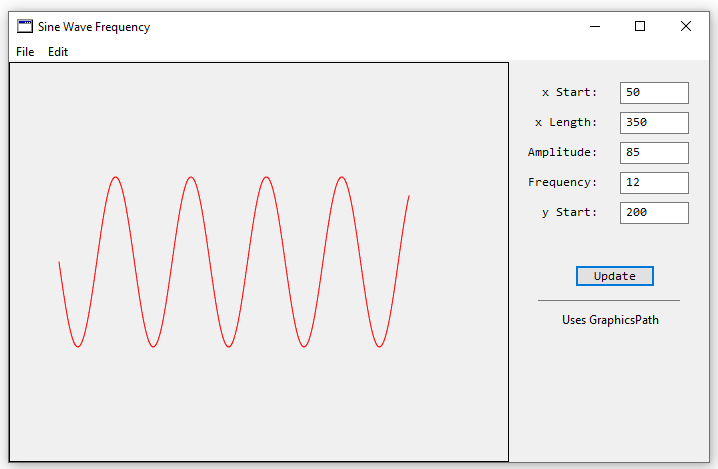With the new Xojo 2020 r2 version, there have been a few changes for 0-based drawing and using the GraphicsPath technique. This example incorporates both changes into a modified method to work with these changes, and the output is shown below.

*Uses Xojo API2There are few visible changes to the user, and the changes to code in the DrawWavyLine2 method are to change the range of the For-Next loop. Next is the addition of the GraphicsPath method which appears to draw data in memory and then has a single call to g.DrawPath to make a single update to the Canvas.

Here is a listing of the modified method:

 Public Sub DrawWavyLine2(g as Graphics, XStart as Double, XLength as double, Amp as double, Frequency as Double, YStart as Double) //This uses the Graphics path method to draw the sine wave Var i as integer Var gp as New GraphicsPath For i = 0 to XLength-1 g.ForeColor = RGB(255,0,0) //Red gp.MoveToPoint(i+XStart, Amp*sin((1/Frequency)*(i))+YStart) gp.AddLineToPoint(i+1+XStart, Amp*sin((1/Frequency)*(i+1))+YStart) Next i g.DrawPath gp //Draw a border g.ForeColor = RGB(0,0,0) //Black g.DrawRect(0,0,g.Width, g.Height)End Sub

GraphicsPath requires two lines of code to draw which are repeated in a For-Next Loop and once the lines have been drawn then a single call to draw to the Canvas occurs with g.DrawPath gp.